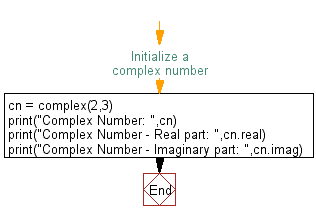﻿ Python Math: Print a complex number and its real and imaginary parts - w3resource# Python Math: Print a complex number and its real and imaginary parts

## Python Math: Exercise-32 with Solution

Write a Python program to print a complex number and its real and imaginary parts.

Sample Solution:-

Python Code:

``````#Initialize a complex number
cn = complex(2,3)
print("Complex Number: ",cn)
print("Complex Number - Real part: ",cn.real)
print("Complex Number - Imaginary part: ",cn.imag)
```
```

Sample Output:

```Complex Number:  (2+3j)
Complex Number - Real part:  2.0
Complex Number - Imaginary part:  3.0
```

Flowchart:## Visualize Python code execution:

The following tool visualize what the computer is doing step-by-step as it executes the said program:

Python Code Editor:

Have another way to solve this solution? Contribute your code (and comments) through Disqus.

What is the difficulty level of this exercise?

Test your Programming skills with w3resource's quiz.

﻿

```>>> students = [{'name': 'John', 'score': 98}, {'name': 'Mike', 'score': 94}, {'name': 'Jennifer', 'score': 99}]